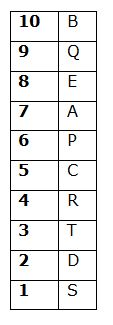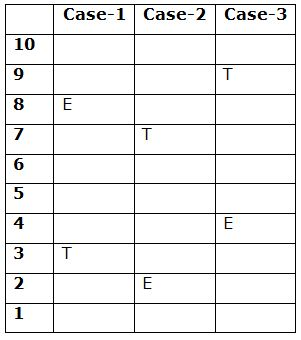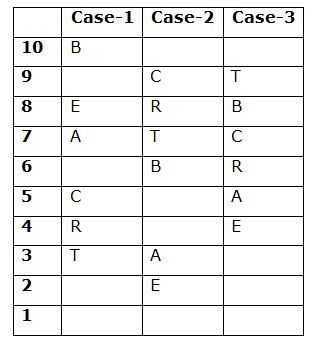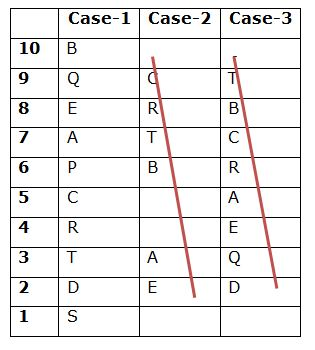# CWC/FCI Prelims 2019 Reasoning Ability Questions (Day-26)

Dear Readers, Exam Race for the Year 2019 has already started, To enrich your preparation here we are providing new series of Practice Questions on CWC/FCI Prelims 2019 Reasoning Ability Questions on daily basis. Aspirants, practice these CWC/FCI Prelims 2019 Reasoning Ability Questions (Day-20) on a regular basis to improve your score in the aptitude section. Start your effective preparation from the right beginning to get success in the upcoming CWC/FCI Exam.

[WpProQuiz 5734]

Direction (1-5): Read the following information carefully and answer the questions given below.

Ten boxes A, B, C, D, E, P, Q, R, S and T are kept one above another in a stack. The stacks are numbered as the bottom most stack is numbered 1, the above one is numbered 2 and so on the topmost stack is numbered 10.

Only four boxes are kept between the box E and T, which is kept at one of the odd numbered position but not at the bottom most. The box B kept above the box E. Only two boxes are kept between the box A and the box B, which is kept above A. The box B does not kept at odd numbered position. As many boxes kept above the box A is same as below the box R, which is does not kept at topmost position. The box C is kept immediately above the box R.As many boxes kept between R and Q is same as kept between A and D. Neither the box Q nor D is kept at the topmost or the bottommost position. The box D does not kept at the odd numbered position. Not more than one box kept between the box A and the box P.

1) How many boxes are kept between the boxes T and D?

a) None

b) One

c) Two

d) Three

e) More than three

2) Which of the following represent the boxes which are kept at the topmost and the bottom most positions?

a) S, Q

b) Q, R

c) A, R

d) B, S

e) None of these

3) Four of the following five are alike in a certain way and hence form a group. Which one of the following that does not belong to the group?

a) D

b) S

c) Q

d) C

e) A

4) If E is related to T and S is related to B in a certain way. Then, C is related to which of the following?

a) D

b) P

c) Q

d) R

e) S

5) Which of the following statements is true?

a) The box E kept immediately above the box Q

b) Only one box is kept below the box D

c) None of the box kept between C and T

d) More than three boxes are kept between E and R

e) None is true

Direction (6-10): Read the following information carefully and answer the questions given below.

H 4 7 \$ 2 P W 5 E M % @ 8 # 3 A J & U G R 6 4 * ? B 9 T

6) How many such symbols are there each of which is preceded by a number and followed by a letter?

a) None

b) One

c) Two

d) Three

e) More than three

7) How many such consonants are there each of which is immediately preceded by a letter and immediately followed by a symbol?

a) None

b) One

c) Two

d) Three

e) More than three

8) If all the symbols are removed from the above arrangement, then what will be the thirteenth element from the right end?

a) M

b) 8

c) E

d) J

e) U

9) If first element is interchanged with the fifteenth element, second element is interchanged with the sixteenth element and so on till the last. Then, which of the following will be the fifth to the left of \$?

a) T

b) H

c) B

d) 9

e) #

10) Four of the following five are alike in a certain way and hence form a group. Which of the following one that does not belong to the group?

a) %#8

b) U6R

d) WME

e) 7P2

Direction (1-5):• Only four boxes are kept between the box E and T, which is kept at one of the odd numbered position but not at the bottommost. The box B kept above the box E.• Only two boxes are kept between the box A and the box B, which is kept above A. The box B does not kept at odd numbered position. As many boxes kept above the box A is same as below the box R, which is does not kept at topmost position. The box C is kept immediately above the box R.• As many boxes kept between R and Q is same as kept between A and D. Neither the box Q nor D is kept at the topmost or the bottom most position. The box D does not kept at the odd numbered position. Not more than one box kept between the box A and the box P.
• So, Case-2 and Case-3 will be dropped.Direction (6-10):

H 4 7 \$ 2 P W 5 E M % @ 8 # 3 A J & U G R 6 4 * ? B 9 T

There is no such symbol.

H 4 7 \$ 2 P W 5 E M % @ 8 # 3 A J & U G R 6 4 * ? B 9 T

H 4 7 2 P W 5 E M 8 3 A J U G R 6 4 B 9 T